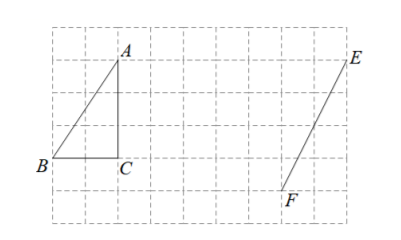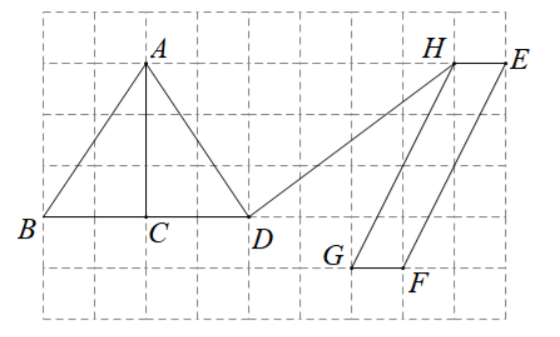(1) 在方格纸中面出 $\triangle A D C$, 使 $\triangle A D C$ 与 $\triangle A B C$ 关于直线 $A C$ 对称 (点 $D$ 在小正方形的顶点上);
(2) 在方格纸中画出以线段 $E F$ 为一边的平行四边形 $E F G H$ (点 $G$, 点 $H$ 均在小正方形的顶点上), 且 平行四边形 $E F G H$ 的面积为 4 . 连接 $D H$, 请直接写出线段 $D H$ 的长.
【答案】 (1)(2) DH=5